# Electric Field

Point charges exert an electrostatic force on one another, even if they are not in contact or near to one another. This occurs through the presence of an electric field around every charge.

An electric field is a region of space in which an electric charge will experience a force. Electric fields, like magnetic fields, have direction. The direction is determined by the direction in which a positive charge would move if placed in the field.

Electric field lines can be used to show the strength and direction of an electric field at a point, much like magnetic field lines are used to represent the magnetic fields around magnets.

Theoretical lines, drawn perpendicularly to the charge, are used to represent the strength and direction of the electrostatic force. The closer the lines are together, the stronger the electrostatic force. The greater the charge, the stronger the electrostatic force (from Coulomb's Law), and the greater the number of electric field lines that are drawn around the charge. The electric field lines point away from positive charges and toward negative charges; this is indicated by arrows on the field lines.Electric field around two unlike charges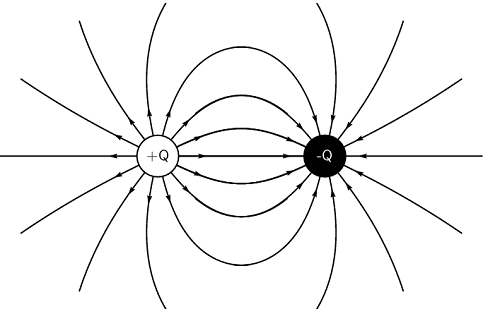Electric field around two like charges - positive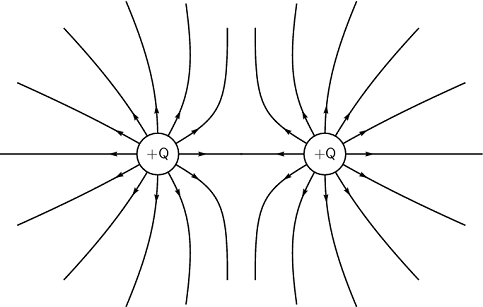Electric field around two like charges - negative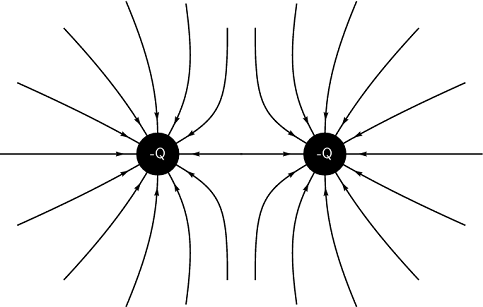Electric field around two charges of different magnitude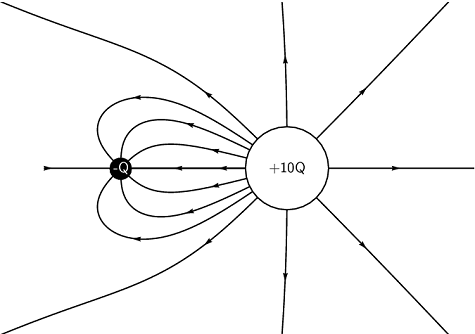The size or magnitude of the electric field € can be reflected as the electrostatic force (F) per unit charge (q). This can be expanded by substituting in the formula for Coulomb's law (F =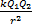), where k is the electrostatic constant,Q1 and Q2are point charges, and r is the distance between them:

E ==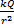Examples.

• Calculate the electric field strength 30 cm from a 5 nC charge.

• E =E =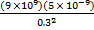E = 4.99 x 102 N.C-1

• Two charges of Q1 = -6 pC and Q2 = -8 pC are separated by a distance of 3 km. What is the electric field strength at a point that is 2 km from Q1 and 1 km from Q2? The point lies between Q1 and Q2.

In order to determine the magnitude of the resultant electric field, it is necessary to determine the electric field of each separately and then combine them.

For Q1:

E =E =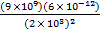E = 41.3 x 10-8 N.C-1

For Q2:

E =E =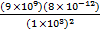E = 7.1 x 10-8 N.C-1

Since the two charges are both negative, the charges repel one another and the electric fields are in opposite directions. This means one needs to subtract the electric field values since they are working in opposite directions to the point.

Etotal = 1.3 x 10-8 - 7.1 x 10-8

Etotal = -5.8 x 10-8 N.C-1 in the direction of the -8 pC charge as it has the stronger electrostatic force.

To summarise, an electric field is an area where an electric charge will experience a force. Electric fields can be represented using field lines and the magnitude of the electric field is defined as the force per unit charge.